# Orthogonal

## Riemannian Thermodynamics [Extra]

Orthogonal

### The Riemannian Black Body Spectrum

The black body spectrum describes the distribution of energy between light of different frequencies in thermal radiation — the radiation created when an object’s thermal energy is freely exchanged with light. We’re not going to try to calculate any of the properties of different kinds of matter in the Riemannian universe that would determine their propensity to exchange energy with the electromagnetic field; in what follows, we’re simply going to assume a context in which energy can move freely between light of different frequencies, as it would in the presence of the kind of perfect emitter and absorber of radiation as is usually assumed when analysing the same kind of situation in our own universe.

We’ll start by considering the electromagnetic field within some kind of enclosure or cavity, with completely opaque walls and the field in thermal equilibrium with the material that surrounds it. Following the treatment of the Lorentzian case by Reif , we will assume that the precise boundary conditions imposed on the field aren’t important. For the sake of simplicity we’ll examine a cubical enclosure, with side length L and volume V = L3, on which we impose periodic boundary conditions: the field at any face of the cube will agree with its value at the corresponding point on the opposite face.

Any solution of the Riemannian Scalar Wave equation meeting these boundary conditions can be written as the real part of a linear combination of plane waves of the form:

fi, j, k(x, y, z, t) = exp(–i ki, j, k · (x, y, z, t))
ki, j, k = (2 π / L)(i ex + j ey + k ez) + ωi, j, k et
ωi, j, k = √[ωm2 – (4 π2 / L 2)(i2 + j2 + k2)]

where i, j and k are integers. They can be positive, negative or zero, but the need for the expression within the square root sign in the definition of ωi, j, k to be positive restricts the choice to a finite set of possibilities, which corresponds to the restriction on the spatial frequency not to exceed νmax.

Of course we want a vector solution, not a scalar one, and for each triplet of integers we can find three unit vectors orthogonal to the propagation vector ki, j, k and each other, giving us three polarisations for each mode. Explicitly:

f (1)i, j, k(x, y, z, t) = [1/ωm][(2 π / L)(i etj ez + k ey) – ωi, j, k ex] fi, j, k(x, y, z, t)
f (2)i, j, k(x, y, z, t) = [1/ωm][(2 π / L)(–i ezj et + k ex) + ωi, j, k ey] fi, j, k(x, y, z, t)
f (3)i, j, k(x, y, z, t) = [1/ωm][(2 π / L)(–i ey + j ex + k et) – ωi, j, k ez] fi, j, k(x, y, z, t)

Now, suppose we set the four-potential A of the electromagnetic field equal to a linear combination of these vector modes. What will be the total energy in the electromagnetic field? Using the formula we previously derived for the energy density in plane waves, we can show that if:

A = A(1) f (1)i, j, k + A(2) f (2)i, j, k + A(3) f (3)i, j, k

then the total energy in the cubical cavity is:

U = (1/2) V (A(1)2 + A(2)2 + A(3)2) ωi, j, k2

If we add further modes to A with other values for i, j, k, the energies simply add; this is a consequence of the orthogonality of the trigonometric functions with different numbers of wavelengths across the cavity. So a completely general expression for the energy would just be a sum of squared terms like this, including the coefficients for every mode.

[If we wanted to, we could work with standing wave modes instead of plane-wave modes. For every pair of plane-wave modes travelling in opposite directions, f (p)i, j, k and f (p)i, –j, –k, we can form two standing-wave modes, (f (p)i, j, k ± f (p)i, –j, –k)/√2. The exception, where i=j=k=0, is already a standing wave. The total energies of these modes are the same as those of the plane-wave modes.]

How many modes are there with an angular frequency between ω and ω+dω? If we assume the cavity is sufficiently large that i, j and k take very large values, we can get a good approximation to this by treating them as continuous quantities. If we put r2 = i2 + j2 + k2, the count of modes is three times (for the three polarisations) the volume of a spherical shell of radius r and thickness |dr|, where:

ω2 = ωm2 – (4 π2 / L2) r2

So we have the density of modes:

ρ(ω) dω = 3 × 4 π r2 |dr| = 3 V ω √(ωm2 – ω2) / (2 π2) dω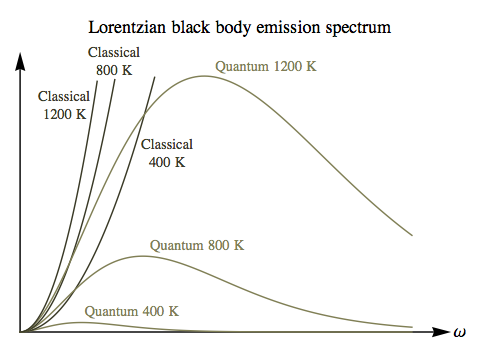The correct treatment of the black body spectrum is quantum mechanical, of course, and we will derive that version of the spectrum shortly, but it’s worth going through a purely classical calculation first. In the Lorentzian universe, because there is no upper limit on the frequency of light the total number of modes in any cavity is infinite. Before quantum mechanics was developed this was very puzzling, because a result in classical statistical mechanics known as the equipartition theorem suggested that every mode of this kind should have an equal share of the total energy, which made no sense if there was an infinite number. So there was no satisfactory classical calculation of the black body spectrum at high frequencies. In the Riemannian universe, the finite number of modes gives a reasonable classical result across the spectrum.

The equipartition theorem states that in a system at equilibrium with temperature T, every degree of freedom of the system that contributes to the total energy with a term proportional to the square of a phase space coordinate or momentum has an average energy of (1/2) k T, where k is Boltzmann’s constant, which just sets the temperature scale. However, although our individual cavity modes each look like a single coordinate, they really involve both a coordinate and a momentum: the instantaneous amplitude of the mode, which is oscillating at a frequency of ωi, j, k, and its rate of change, which is the corresponding momentum. Just as in the case of a harmonic oscillator (such as a mass on a spring) where the potential energy is proportional to the displacement of the oscillator, squared, and the kinetic energy is proportional to the time rate of change of the displacement, squared, the sum of the two energies is constant, but if we’re considering a system of such oscillators at thermal equilibrium, each oscillator has an average energy of k T, not (1/2) k T.

So the energy density of the field in the cavity, at a given frequency ω, will be:

uclassical(ω) dω = 3 k T ω √(ωm2 – ω2) / (2 π2) dω

The classical energy density spectrum will always have a peak at:

ωpeak = ωm / √2 ≈ 0.707 ωm

regardless of the temperature. The total energy density across the spectrum is the integral of uclassical(ω) for ω from 0 to ωm:

uclassical, total = ωm3 k T / (2 π2)

In the Lorentzian case, the shape of the spectrum of the radiation emitted from a cavity is exactly the same as the shape of the spectrum of the radiation within the cavity, because any radiation that escapes through a small aperture will all be travelling at a single speed, the speed of light. But in the Riemannian universe, each frequency of light will travel at a different speed, making the black body emission spectrum quite different from the distribution of energy within the cavity itself.

To compute the emitted power per unit area from a black body, as well as multiplying by the velocity for each angular frequency:

v(ω) = √(ωm2 – ω2) / ω

we need to integrate over all spatial directions for the radiation. For a given aperture with a specific orientation, this gives a further factor of 1/4. So we have:

Iclassical(ω) dω
= (1/4) v(ω) uclassical(ω) dω
= 3 k Tm2 – ω2) / (8 π2) dω

So the classical emission spectrum is just an upside-down parabola, with a peak at ω=0. The only effect of the temperature is to increase the overall power emitted, without changing the shape of the spectrum at all. This is certainly in sharp contrast with the black body spectrum in our own universe, where light from a hotter source has its frequency peaking at shorter wavelengths than light from a cooler source — and the total power emitted scales with the fourth power of the temperature, rather than the linear scaling we’ve found here.

Of course our Riemannian calculations so far have been classical, and to make a true comparison we need the quantum version. We expect Riemannian light to obey the same general laws of quantum statistics as photons in our own universe, that of bosons (specifically, spin-1 particles) that are uncharged and hence are their own antiparticle. Each Riemannian photon of frequency ν has an energy hν, where h is Planck’s constant, or ℏω, where ℏ=h/(2π) and ω is the angular frequency.

The relevant calculations in quantum statistical mechanics  tell us that each mode in the cavity’s field will be occupied, on average, by a number of Riemannian photons that is a function of the energy of the mode and the temperature:

n(ω) = 1 / [exp(E(ω)/(k T)) – 1] = 1 / [exp(ℏω/(k T)) – 1]

For high temperatures (or the limit of small ℏ), this becomes n(ω) ≈ k T / (ℏω), which amounts to asserting exactly the same thing as the equipartition theorem: that each mode will have an average energy of k T. Note that this expression simply makes no sense for negative temperatures: there can be no thermal equilibrium for a system like this at a negative temperature.

Multiplying n(ω) by the energy ℏω and the density of states ρ(ω) gives us the quantum version of the cavity energy density spectrum:

uquantum(ω) dω = 3 ℏω2 √(ωm2 – ω2) / [2 π2 (exp(ℏω/(k T)) – 1)] dω

As with the classical version, we need to multiply by one quarter of the velocity of light of the same frequency to obtain the spectrum for emitted power:

Iquantum(ω) dω = 3 ℏω (ωm2 – ω2) / [8 π2 (exp(ℏω/(k T)) – 1)] dω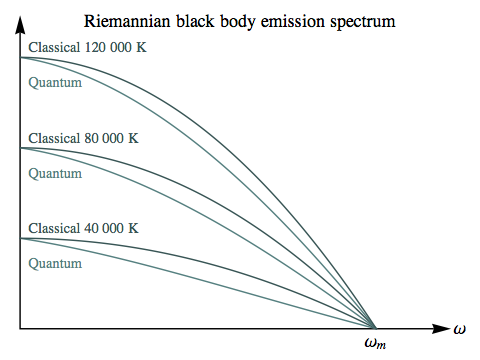In these Riemannian diagrams we’ve set ωm = 2π × 1015 Hz, and then used the real-world values of Planck’s and Boltzmann’s constants. The Riemannian classical and quantum spectra can be seen coming proportionately closer as the temperature increases: the absolute difference at the widest separation is roughly the same, but as the entire spectrum grows larger this represents a smaller fraction of the energy.

In interpreting these plots, it’s important to keep in mind that in the Riemannian universe positive temperatures for most forms of matter involve extreme conditions — such as relativistic velocities in a gas — and the lower the positive temperature, the more extreme those conditions. So higher temperatures here represent the least exotic states.

This suggests that the discrepancy between the quantum and classical black body spectra would be a relatively minor phenomenon in the Riemannian universe, unlikely to play the same role in the development of quantum mechanics that it did in our own history. If you compare the classical/quantum difference here with the earlier plot for the Lorentzian case, the Lorentzian ultraviolet catastrophe is unmissable, but the Riemannian “blue sag” is unspectacular, and would require accurate measurements across the entire spectrum to be detected at all.

However, there is a different kind of “catastrophe” associated with cavity radiation: the mean pressure of the radiation, at any temperature, is infinite! Why? For cavity modes with an angular time frequency ω close to zero, their amplitude A needs to grow increasingly large in order for their energy, (1/2) V A2 ω2, to be equal to the uniform value k T determined by the equipartition theorem. But while their energy is kept bounded by the low value of ω, their spatial frequency is close to ωm, so their momentum and pressure, which are also proportional to A2, are unbounded.

Of course perfect black bodies, and perfect thermal equilibrium, are just idealisations, so this doesn’t show that any real physical quantity would become infinite. But it does make it clear that the radiation pressure in any system where light can freely exchange energy between every possible mode will be extremely high.

### The Single-Particle Partition Function

The states we have enumerated for cavity radiation in the previous section are essentially the states available to any Riemannian particle. The factor of three for the three polarisations might be replaced by a different factor, but apart from that (which will depend on the particle’s spin), and the value of ωm (which will depend on the particle’s mass), the method of counting the possible states of a single particle will be the same. Of course in the case of bosons there can be any number of particles occupying each state, while in the case of fermions there can be at most one, but the initial step of identifying the states themselves is no different between bosons and fermions.

When dealing with systems in thermal equilibrium with a specified temperature, it’s useful to package the information we have about the states of the system into something called the partition function (see also ). Its definition in general is:

Z = Σr exp(–β Er)
where β = 1/(k T)

The index r is simply a label for the states of the system, and Er represents the energy of each state. We’ve defined a temperature parameter β which has units of inverse energy, and can be more useful to work with than temperature itself. β is zero for infinite temperature and goes to infinity in positive and negative directions as the temperature approaches zero from above and below.

Each term in the partition function is proportional to the probability of finding the system in one of its states. The probability itself can be found by dividing that term by the sum of all the terms, which is the partition function itself. So:

P(r) = Probability of finding system in state r = exp(–β Er) / Z

One of the most useful things about the partition function is that various average properties of the system can be extracted from it quite easily. For example, we can find the mean energy of the system as follows:

Emean = Σr P(r) Er
= [Σr exp(–β Er) Er] / Z
= –[∂β Z] / Z
= –∂β ln Z

The partition function for a single, spinless Riemannian particle with mass m (and hence maximum angular frequency m/ℏ) confined to a volume V is:

Zm = Σi, j, k exp(–β Ei, j, k)
= Σi, j, k exp(–β ℏ ωi, j, k)
= Σi, j, k exp(–β ℏ √[(m/ℏ)2 – (4 π2 / V2/3)(i2 + j2 + k2)])

where the sum is over all integers i, j, k, both positive and negative, for which the expression in the square root sign is non-negative. We’ve adapted the expression for ωi, j, k from the previous section to make use of the particle mass m rather than the maximum angular frequency for the particle in question, and replaced the cavity side length L with V1/3. In the limit of a large number of states we ought to be able to approximate this as an integral over a spherical region in (i, j, k) space, which we can describe most easily in spherical polar coordinates with r2 = i2 + j2 + k2:

Zm = 4 π ∫0R exp(–β ℏ √[(m/ℏ)2 – (4 π2 / V2/3) r2]) r2 dr
where R = V1/3 m / (2 π ℏ) is the value for r at which the energy is 0.

With the change of variable r = R s this becomes:

Zm = 3 Z001 exp(–β m √[1 – s2]) s2 ds
where Z0 = (4/3) π R3 = V m3 / (6 π23) is the total number of states.

We can make a further change to an angular variable γ, with s = sin(γ), to obtain:

Zm = 3 Z00π/2 exp(–β m cos(γ)) sin(γ)2 cos(γ) dγ

This last form can be interpreted as an integral over the half-3-sphere on which the particle’s energy-momentum vector must lie.

Amazingly enough, it’s possible to write an explicit formula for this integral:

 Single-Particle Partition Function Zm = Z0 [1 + 3 π [L2(β* ) – I2(β* )]/(2β*)] where Z0 = V m3 / (6 π2 ℏ3) and β* = β m (Riemannian)Here L2 is a modified Struve function and I2 is a modified Bessel function of the first kind.

The plot on the right shows the partition function, along with a quadratic approximation that’s suitable for small values of β, which correspond to very high temperatures.

limβ→0 ZmZ0 [1 – 3 π β*/16 + β*2/5]

Of course there’s nothing to stop us taking a power series to a higher order if we wish. There’s also an asymptotic form suitable for large negative values of β, which correspond to small negative temperatures. This approximation begins to become useful once β*<–4.

limβ→–∞ Zm
[3 Z0 / β*2] [1 – exp(–β*)(8β*+15) / (8 √(–2β*/π))]

### Ideal Gases

#### Dilute Gas With a Fixed Number of Particles

Suppose we have a gas consisting of a fixed number, N, of particles, sufficiently dilute that the average number of particles in any quantum state is much less than 1. In this case, it will be irrelevant whether the particles are bosons or fermions, and we have a kind of classical limit. However, the particles are still correctly considered indistinguishable, which avoids some problems that appear in a truly classical treatment.

In this scenario, we can describe the partition function for the gas as a whole, which we’ll write as ZG, in terms of the partition function for a single particle that we derived in the previous section. Making use of some general calculations from Reif , we have:

ln ZG = NN ln N + N ln Zm

We’ve given the partition function in terms of its natural logarithm, as that’s the form that’s most directly useful when computing various macroscopic properties of the gas.

##### Energy

The first quantity we’ll compute is the mean energy of the gas:

Emean = –∂β ln ZG
= –Nβ ln Zm
= –N [∂β Zm] / Zm
= 3 N m (1/β* + [π L1(β*) – π I1(β*) + 2] / [3 π I2(β*) – 3 π L2(β*) – 2 β*])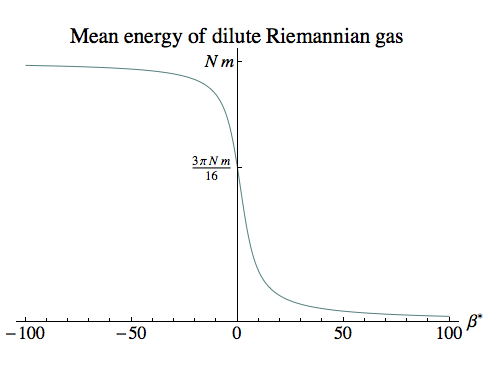where β* = β m. The infinite-temperature limit can be found easily from our quadratic approximation to the partition function:

limβ→0 Emean = 3 π N m / 16 ≈ 0.589 N m

As the plot on the right shows, for large negative values of β (which correspond to small negative temperatures) the particles are moving slowly, and the average energy is close to the sum of their rest-mass energies.

For small values of β (which correspond to large temperatures) the particles are moving faster, and the total energy is less — just as we expect from the relationship between total energy and kinetic energy.

And for large positive values of β (which correspond to small positive temperatures), the gas is in a highly relativistic state where the kinetic energy is close to the rest mass, reducing the total energy almost to zero.

##### Pressure

Next we will compute the average pressure of the gas. The usual expression for the pressure in terms of the partition function  makes use of the fact that when a system’s energy depends on its volume, the rate of change of energy with respect to volume is the opposite of the pressure exerted by the system. (Imagine the system slowly expanding, doing work against a restraining force. The work done is force times the linear distance of the expansion, or pressure times the volume change.)

pmean = Σr P(r) pr
= [Σr exp(–β Er) pr] / Z
= [Σr exp(–β Er) (–∂V Er)] / Z
= [∂V Σr exp(–β Er)] / [β Z]
= [∂V ln Z] / β

However, this is not correct for the Riemannian case! One minor difference is that in Riemannian physics, the rate of change of energy with volume is the pressure itself, rather than its opposite; this is essentially for the same reasons that the momentum that is conjugate to each spatial coordinate in the Hamiltonian treatment of a free particle is the opposite of the usual momentum, as we discussed previously.

But even if we change the sign here, we’re still left with an equation that — although it looks familiar — actually makes no sense.

Let’s go through with the calculation anyway, to see where the problems arise. Because Zm is just a linear function of V (via Z0), we have:

V ln Zm = [∂V Zm] / Zm = (Zm / V) / Zm = 1 / V

Turning to the partition function for the whole gas, and using the standard pressure formula with a change of sign for the Riemannian energy/volume/pressure relationship, we get:

pmean = –[∂V ln ZG] / β
= –N [∂V ln Zm] / β
= –N / (β V)   [Wrong!]

which is equivalent to:

pmean V = –N k T   [Wrong!]

This is just the standard equation of state for an ideal gas, with a change of sign. Now, while that’s about what we’d expect for low negative temperatures, and it might even seem superficially plausible that the pressure ought to become infinite when the temperature becomes infinite ... this equation implies that once we crossed into positive temperatures, the pressure would become negative! Amusing as it would be to live in a world where bombarding the walls of a container with relativistic particles put the container under tension rather than pressure, that would make no physical sense at all.

The resolution of this puzzle is that our partition function Zm carries an extra dependence on the volume of the container that isn’t present at all in the Lorentzian physics under which the standard formula for the pressure in terms of the partition function was derived. When we change the volume of the container, we don’t just change the energy levels of the various particle states, we also change the number of states. A larger container, in the Riemannian case, allows a greater finite number of particle states before the minimum wavelength is reached. In the Lorentzian case, because there is no minimum wavelength there is always an infinite number of states.

The simplest cure for this is to go back to one of our earlier forms for Zm:

Zm = 4 π ∫0R exp(–β ℏ √[(m/ℏ)2 – (4 π2 / V2/3) r2]) r2 dr
where R = V1/3 m / (2 π ℏ)

From this we can identify — and subtract out — the effect of V on the upper limit of the integral here (via R), and leave behind only the effect we want: the change in the energy levels. The term in ∂V Zm due to the integral limit is just the integrand (including the overall constant of 4 π) evaluated at r = R, multiplied by ∂V R. This comes to:

4 π exp(0) R2 × (1/3) V–2/3 m / (2 π ℏ)
= (4/3) π R3 / V
= Z0 / V
where Z0 = (4/3) π R3 = V m3 / (6 π23)If we repeat our original calculation, but subtract this spurious contribution from ∂V Zm to leave only the average pressure, we get:

pmean = –N [(Zm / V) – (Z0 / V)] / (Zm β)
= –N / (β V) [1 – Z0 / Zm]

which is equivalent to:

pmean V = –N k T [1 – Z0 / Zm]   [Right!]

When the temperature is negative, Zm > Z0, so the correction factor in square brackets is positive and hence the pressure is positive. Indeed, for low negative temperatures Zm is extremely large, so the correction factor is very close to 1, and we have the traditional ideal gas law, adjusted for the sign of T.

But when the temperature is positive, Zm < Z0, the correction factor is negative, and the pressure remains positive.

The pressure is finite at infinite temperature. Using our quadratic approximation for Zm when β is close to zero, it’s easy to show that:

limβ→0 pmean = 3 π N m / (16 V)

As β goes to positive infinity, the temperature approaches zero from above, heading into the realm of ever more extreme relativistic particle velocities, and the pressure goes to infinity, as you’d expect.

##### Entropy

The entropy of a system can also be found from the partition function :

S = k (ln Z + β Emean)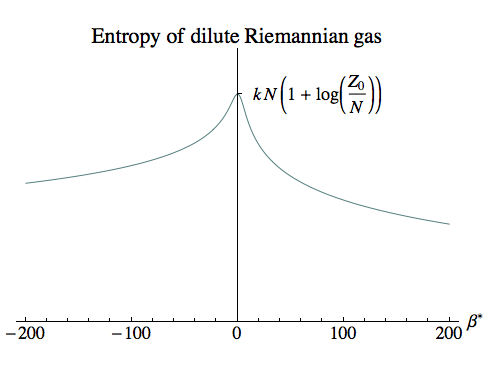For our dilute gas we have:

SG = k (ln ZG + β Emean)
ln ZG = NN ln N + N ln Zm
Emean = –N [∂β Zm] / Zm

We won’t write out the entropy in terms of all the logs, Bessel and Struve functions, but as the plot shows it has a finite peak at β=0, where the temperature is infinite.

How are the N particles distributed by energy, at different temperatures? On our assumption of a dilute gas, the average number of particles in any state labelled r can be shown to be :

nr = N exp(–β Er) / [Σs exp(–β Es)]
= N exp(–β Er) / Zm

We’re interested in the distribution of particles over energy, E = ℏω, so we can make use of the density of states over ω we computed for cavity radiation, dropping the factor of 3 for the polarisations since we’re imagining a spinless particle for our ideal gas.

ρ(ω) dω = V ω √(ωm2 – ω2) / (2 π2) dω
ρ(E) dE = V E √(m2E2) / (2 π23) dE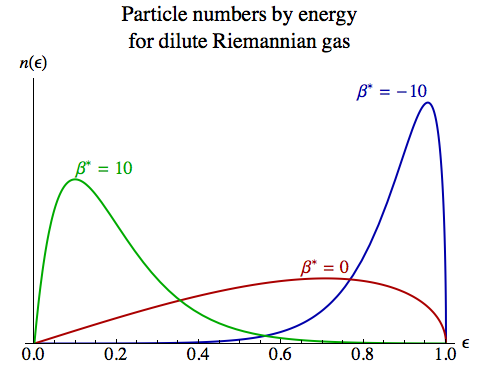We multiply this by the average occupancy of each state at this energy, to obtain:

n(E) dE
= N [V / (2 π23)] E √(m2E2) exp(–β E) / Zm dE
= N [3 Z0 / (m3 Zm)] E √(m2E2) exp(–β E) dE

It’s more convenient to deal with energy as a multiple ε of the particle mass m, so E = ε m:

n(ε) dε = N [3 Z0 / Zm] ε √(1 – ε2) exp(–β* ε) dε

The diagram on the right shows the distribution for three values of β*. At lower temperatures — both positive and negative — the particles’ energies are relatively constrained, while at infinite temperature they’re spread more widely.

The infinite temperature dilute gas possesses an interesting property: its stress-energy tensor is SO(4)-invariant, so any observer moving within such a gas at any speed will measure exactly the same pressure and energy density for their surroundings. We can see this by noting that the mean energy density and the mean pressure of the gas, at infinite temperature, are both equal to 3 π N m / (16 V). It follows that the stress-energy tensor is simply a multiple of the identity matrix, and will be unaltered by a change of coordinates.

Looking closely at the distribution of particles by energy, though, shows that this SO(4)-invariance is achieved in a rather subtle fashion. The distribution for β=0, or infinite temperature, is:

n(ε) dε = 3 N ε √(1 – ε2) dε

If we switch to an angular variable, γ, equal to the angle between the particles’ 4-velocity and the time axis, then ε=cos(γ) and we have:

n(γ) |dγ| = 3 N cos(γ) sin(γ)2 |dγ|

The rotationally invariant measure on a 3-sphere, after integrating out all the other coordinates concerned with different directions in three-space, is just sin(γ)2 dγ, so this tells us that the particles’ 4-velocities are not distributed uniformly on the 3-sphere, as we might have expected for an SO(4)-invariant state. Compared to a uniform distribution, the particles are preferentially distributed towards lower velocities, closer to the time axis, where cos(γ) is larger.

How, then, does the infinite-temperature gas get to be SO(4)-invariant? If we examine a particle with energy E = m cos(γ), the time component ut of its 4-velocity u will be cos(γ). If the energy density in the particle’s rest frame is ρ, its stress-energy tensor is ρ uu. This means the particle’s contribution to the total energy in the stationary container’s frame is V ρ (ut)2 = V ρ cos(γ)2. Equating this with E = m cos(γ) gives a rest-frame energy density that is increased as the particle’s speed rises and cos(γ) falls:

ρ = m / (V cos(γ))

This factor might seem puzzling, because measured in the moving particle’s rest frame the width of the container is increased; this is the Riemannian counterpart of the more familiar Lorentzian relativistic length contraction. But by the same token, if several particles are moving across the container with the same velocity, in their shared rest frame they will be measured as being closer together than they would by an observer at rest with respect to the container.

So for an observer moving rapidly through the gas, the relative paucity of particles with low values of cos(γ) is compensated for by the fact that they’ll appear more closely packed together. Though the distribution of the particles’ velocities won’t look the same, the overall energy density (and pressure) will be unchanged.

#### Fermion Gas With Pair Production

For a gas of slow-moving particles, it’s natural to assume that their number will remain fixed. But when the particles are moving at relativistic speeds, it’s a rather artificial constraint, because random high-speed collisions might sometimes scatter particles into the past (a kind of collision we’ve looked at previously). Whether this requires the presence of antiparticles will depend on the nature of the particle (some particles will be their own antiparticle, as photons are in our universe), but it will certainly involve changes in the total number of particles.

Suppose we have an ideal gas comprised of particles with a spin of ½. They will be fermions, meaning that they obey Fermi-Dirac statistics: each quantum state will be occupied by at most one particle. Let’s assume that a mechanism exists for pairs of particles and antiparticles to be created, with the energy required to do so being balanced by changes in the kinetic energy of the gas. We won’t be interested here in the details of the pair production and annihilation processes; rather, our aim is to discover whether such a system can be in thermodynamic equilibrium in both the positive and negative temperature regimes. In our treatment of cavity radiation, we found that a system of an indefinite number of freely created bosons would always have a positive temperature. Will our fermion gas be the same, due to its ability to create new particles?

Because particles and antiparticles are created and destroyed in pairs, the difference between their numbers will remain constant. We will call the fluctuating numbers of particles and antiparticles NP and NA respectively, and the fixed excess of particles N:

N = NPNA

Every individual quantum state r available to a particle in our container will be occupied by either 0 or 1 particles and either 0 or 1 antiparticles; the Pauli Exclusion principle prohibits there being more than one particle of each kind in each state, but does not prevent a particle and an antiparticle from being in the same state. We will write nP, r and nA, r for the number of particles and antiparticles respectively in the state labelled r.

A state for the whole gas consists of an assignment of 0 or 1 to every nP, r and nA, r, such that the total excess of particles over antiparticles is N. We’ll write ZG(N) for the partition function for a gas with a specific value for N:

ZG(N) = ΣnP, r, nA, r : NPNA = N exp(–β Σr [Er (nP, r + nA, r)])

Here the outer sum is over all states of the gas that have a particle excess of N, and we compute the total energy of each such state of the gas as the sum of the energies of all the individual particles.

There is a finite number of single-particle states in a given container of volume V; we found this number, Z0, when computing the single-particle partition function, though to be precise here we should use twice that value, to account for the two spin states of our fermions:

Nmax = 2 × Z0 = V m3 / (3 π23)

If we have Nmax available states for particles and the same number again for antiparticles, the count of states with an excess of N particles can be found as a sum over the number of antiparticles:

|{nP, r, nA, r : NPNA = N}|
= Σi=0NmaxN Binomial(Nmax, N+i) Binomial(Nmax, i)
= Binomial(2 Nmax, Nmax+N)
≈ [22 Nmax / √(π Nmax)] exp(–N 2 / Nmax)

Suppose we hold β fixed and just think about the way the gas’s partition function ZG(N) varies with N. The number of terms in the outer sum is given by the Gaussian function derived above. The average value of the individual terms in the sum won’t vary rapidly with N, since whatever the value of N they will be sampling all the different states’ energies in much the same way. So we can, very roughly, say that ZG(N) itself will behave like a multiple of the Gaussian that counts the number of terms, i.e.

ZG(N) ≈ C(β) exp(–N 2 / Nmax)

where the constant C(β) depends on β and we’ve absorbed the other factors that depend on Nmax into it.

Now, if we multiply ZG(N) by an exponential function in N, exp(α N), that will displace the peak of the Gaussian:

ZG(N) exp(α N) ≈ C(β) exp(–N 2 / Nmax + α N)
= C(β) exp(Nmax α2/4) exp(–(NNmax α/2) 2 / Nmax)

If we want this function to peak at, say, N0, we set:

α = 2 N0 / Nmax

We can approximate the partition function ZG(N0) by taking a sum without constraints on N and using the location of the Gaussian’s peak to single out the value N = N0:

ΣN=0Nmax ZG(N) exp(α N) ≈ ZG(N0) exp(α N0) ΔN

where ΔN is a measure of the width of the peak. We call the sum on the left-hand side the “grand partition function” ; it is a sum over all possible states of the gas, regardless of particle numbers, modified by an extra term in each energy. Thanks to the lack of constraints, we can go quite far in evaluating it:

ZGRAND = ΣN=0Nmax ZG(N) exp(α N)
= ΣnP, r, nA, r exp(–β Σr [Er (nP, r + nA, r)]) exp(α N)
= ΣnP, r, nA, r exp(–β Σr [Er (nP, r + nA, r)]) exp(α Σr [nP, rnA, r])
= ΣnP, r, nA, r exp(–Σr [nP, rEr – α) + nA, rEr + α)])
= Πr [ (ΣnP, r=0,1 exp(–nP, rEr – α))) (ΣnA, r=0,1 exp(–nA, rEr + α))) ]
= Πr [ (1 + exp(–(β Er – α))) (1 + exp(–(β Er + α))) ]

We can approximate the log of the partition function of the gas as:

ln ZG(N0) ≈ –α N0 – ln ΔN + ln ZGRAND
≈ –α N0 + Σr [ ln (1 + exp(–(β Er – α))) + ln (1 + exp(–(β Er + α))) ]

With some further calculations similar to those in , we can show that the average occupancy numbers for each state are, for particles and antiparticles respectively:

nP, r, mean = 1 / [exp(β Er – α) + 1]
nA, r, mean = 1 / [exp(β Er + α) + 1]

Unlike the case for the Riemannian photon states’ occupancy numbers, these expressions don’t become nonsensical for negative temperatures; indeed for any values of α and β whatsoever they will both lie between 0 and 1, as required for fermions. To find α for a given value of β and N0 we would need to solve:

N0 = Σr (nP, r, meannA, r, mean) = Σr sinh(α) / [cosh(α) + cosh(β Er)]

for α. Since the integral version for this sum doesn’t appear to have a closed analytic form, in general we’d need to solve this numerically. Our initial approximation, α = 2 N0 / Nmax, could serve as a starting point for an iterative solution.

But we can illustrate the main qualitative features of this kind of system if we set N0=0 and consider a gas of precisely equal numbers of particles and antiparticles. By symmetry, we then have α=0 for all values of β, and so:

ln ZG ≈ 2 Σr [ ln (1 + exp(–β Er)]
nP, r, mean = nA, r, mean = 1 / [exp(β Er) + 1]The diagram on the right shows the mean energy of the system, which is roughly the same shape as the mean energy curve for a dilute gas, but involves energies comparable to the particle mass times the total number of single-particle states.

The mean energy was calculated by numerical integration, with the same variables r and s that we used for the single-particle partition function:

Emean = ∫0R (–∂βln ZG) 4 π r2 dr
= 2 ∫0R (–∂βln (1 + exp(–β Er))) 4 π r2 dr
= 6 Nmax m01 s2 √[1 – s2] / (exp(β* √[1 – s2]) + 1) ds

For β*=0 and β*→–∞ the exponential goes to 1 or 0, and the integral can be evaluated exactly, giving 3 π Nmax m / 16 and 3 π Nmax m / 8 respectively.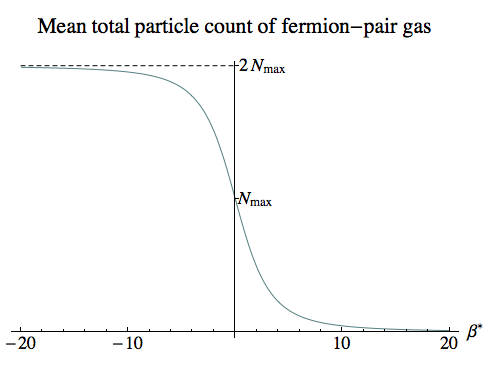This next plot gives the mean total number of particles (including antiparticles). At low negative temperatures the system is close to being saturated, with almost every quantum state filled by both a particle and an antiparticle. At infinite temperatures the states are half-full on average, and the number declines as we move into ever-lower positive temperatures.

Here the integral we’ve plotted is:

Ntotal, mean = ∫0R (nP, r, mean + nA, r, mean) 4 π r2 dr
= 2 ∫0R 1 / (1 + exp(β Er)) 4 π r2 dr
= 6 Nmax01 s2 / (exp(β* √[1 – s2]) + 1) ds

Again for β*=0 and β*→–∞ the exponential goes to 1 or 0, and the integral can be evaluated exactly, giving Nmax and 2 Nmax respectively.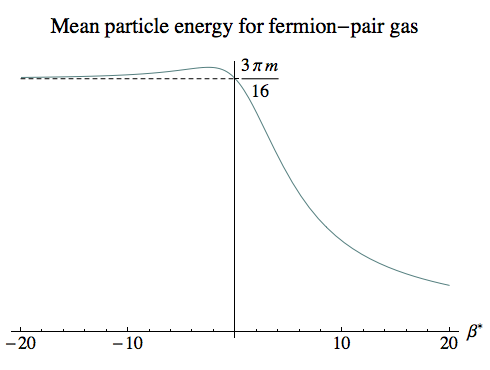The third plot shows the mean energy per particle, and is just the first curve divided by the second. For negative temperatures all the way up to infinity, this remains almost constant at around 3 π m / 16, an average energy over all the states. For small negative temperatures this is because virtually all the states are permanently occupied, while for larger negative temperatures the average occupancy falls but the particles are still spread equally among the states.

It’s only once we move into low positive temperatures that not only do the particle numbers fall, but the particles start to occupy lower energy states. If we were dealing with a system with an excess of N0 particles over antiparticles (or vice versa), then the energy would approach an asymptote where N0 particles filled the lowest N0 energy states. But because we’ve set N0 = 0, the asymptotic energy for low positive temperatures is zero.

It’s important to remember that nothing here tells us about the mechanisms, or the rates, of any pair-production process; these results simply take it for granted that the process exists and has reached equilibrium. The rate at which pair production occurs might well be highly temperature-dependent, and if the rate at some temperature T is very low then the equilibrium state at that temperature might tell us nothing about what we’d actually observe. So we shouldn’t take the existence of these negative-temperature fermion-pair gases — in which almost every quantum state is occupied — as necessarily being the short-term fate of any gas of fermions at a negative temperature!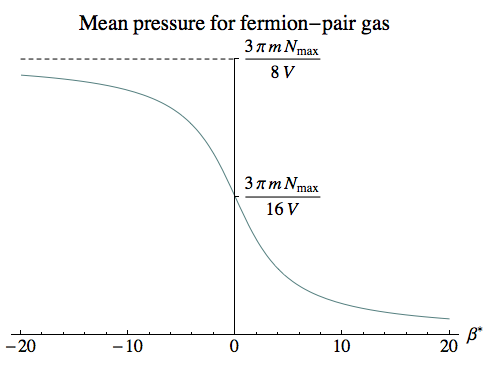However, for a highly relativistic gas with a positive temperature, even classical collisions between particles can lead to changes in particle numbers, so the description here is much more likely to be applicable.

The mean pressure of the system is given by:

pmean = –(1/β) ∂V ln ZG

with the caveat that we need to avoid the pitfall we identified when finding the pressure of a dilute gas and take the derivative with respect to V before integrating over the single-particle states. We’ll omit the details of the calculations.

At infinite temperature, the pressure we find is the same per particle as we found for a dilute gas. So at least at high temperatures, in a situation where the original gas contained N << Nmax particles, the added presence of particle-antiparticle pairs would make a huge difference.

For lower positive temperatures, the number of particle-antiparticle pairs drops faster than the rise in pressure that we saw in the dilute gas due to the greater momentum of each particle. So, curiously enough, the most extreme relativistic gas, with the lowest positive temperatures, would be affected the least by pair production.

### References

 Fundamentals of Statistical and Thermal Physics by Frederick Reif, McGraw-Hill, 1965. Sections 9.13, 9.14, 9.15.

 Reif, op. cit., sections 9.3, 9.5.

 Reif, op. cit., sections 6.5, 6.6.

 Reif, op. cit., section 9.8.

 Reif, op. cit., section 6.5, equation (6.5.12).

 Reif, op. cit., section 6.6, equation (6.6.5).

 Reif, op. cit., section 9.6.Orthogonal / Riemannian Thermodynamics [Extra] / created Wednesday, 6 April 2011